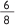# Stage 3 fractions and decimals

Compare and order decimals using strategies such as the number line and diagrams; explain or demonstrate the equivalence of fractions; use estimation to check whether an answer is reasonable; interpret and explain the use of fractions in everyday contexts

## Strategy

Students can:

• compare and order decimals using strategies such as the number line and diagrams
• explain or demonstrate the equivalence of fractions
• use estimation to check whether an answer is reasonable
• interpret and explain the use of fractions in everyday contexts

## Activities to support the strategy

### Activity 1 - equivalent fractions

1. Provide 3 squares of equal size, one divided in half, one divided into quarters and one divided into eighths.

Discuss the number of equal parts in each square. Establish(two over two),(four over four) and(eight over eight) all equal 1 whole.• Fold each square in half and model that(one half) is equal to(two quarters) and(six eighths).
• Using cut up squares model that(one quarter) is equal to( two eighths).and that(three quarters) is equal to(six eighths).
• Discuss the pattern - if the numerator is doubled, so is the denominator.

2. Divide the class into groups and provide each with a variety of shapes divided into fifths, tenths, eighths, quarters and halves. Have groups manipulate the shapes to demonstrate equivalent fractions.

Each group records the sets of equivalent fractions they have modelled, then reports back to the class.

3. Teacher holds up a fraction card, for example ½. Students provide an equivalent fraction for ½. Ask students what has happened to the numerator and the denominator to make the equivalent fraction in each example.

Prompt students to formulate a rule to explain that if the numerator is doubled, so is the denominator.

The rule generated by the students can be placed on a chart in the room.

• Provide students with a worksheet showing a range of shapes divided into different fractions. Students colour a section/sections of each shape.Consider placing divided shapes in different orientations when presenting in modelling or worksheet phase.

Include examples requiring students to:

• colour fifths, tenths, hundredths, eighths, quarters and halves
• colour wholes in the form of, (two over two), (four over two) and so on
• write the equivalent fraction of a shaded shape, for example. if half the shape is shaded the students must fill in the missing numerator(? over two),, (? over 4)(? over 8).Students provide equivalent fractions of a given fraction, for example:

½ equals or(how many quarters?) or(how many eighths?)

Students fill in missing numerators and denominators in mixed format, for example:

½ =(two fourths) =(four eighths).

Repeat activities for thirds, sixths and twelfths.

Extension: When demonstrating halves, quarters and so on in future activities, remember to change orientation of the base shape, by alternating its presentation

### Activity 2 fraction games

These activities involve students working in small groups or pairs to complete a game. Concrete materials can help students to develop a sound understanding of the concepts required when calculating with fractions.

1. Play the game Fraction track as a board game with the whole class, prior to playing it on the computer - Fraction track board game#### Equipment:

• two large game boards of the fraction track
• cards with the fractionstwo post-it notes per team placed at the beginning of the game board (see below)Students play in two teams. The teacher turns over a card and the team decides where to move the post-it note along the fraction track. More than one post-it note can be moved, as long as the total of the fraction card is not exceeded.

For example, when the teacher turns over ½ Tom, Alice and Sean may move:

• one post-it note along by ½
• the first post-it note along byand the second post-it note along by• both notes along byThe first team to move all post-it notes to the end of the track wins.

2. Play the game Coloured Fractions. Coloured Fractions may be played as a whole class activity, in pairs or small groups.

This activity links closely with the Stage 3 Crossing the Wall activity described on page 52 of Fractions, pikelets and lamingtons.

View/print Fractions, pikelets and lamingtons (PDF 4268.57KB)

#### Equipment

• green dice (numerator) labelled:• blue dice (denominator labelled:• one gameboard for 2 studentsTake turns to throw the dice. Colour in the equivalent of the fraction represented by the dice. Record each fraction as the game is played. The first pair to colour the entire game board is the winner.

• At the completion of the game, students add the fractions to check that they total 6.

### Activity 3 -decimals

Students complete activities using a decimal double number line folded along the middle so that only the side showing the end points is visible.

• Place a paper clip on the folded double number line to estimate the position of a given number. Students justify the position for their number.
• Flip over the number line to check their estimation.

For example, ask students to estimate the position of 0.6 using the estimate position worksheet (PDF 24.49KB)

## References

### Australian curriculum

ACMNA127: Find a simple fraction of a quantity where the result is a whole number, with and without digital technologies.

ACMNA125: Compare fractions with related denominators and locate and represent them on a number line.

### NSW syllabus

MA3-7NA: Compares, orders and calculates with fractions, decimals and percentages